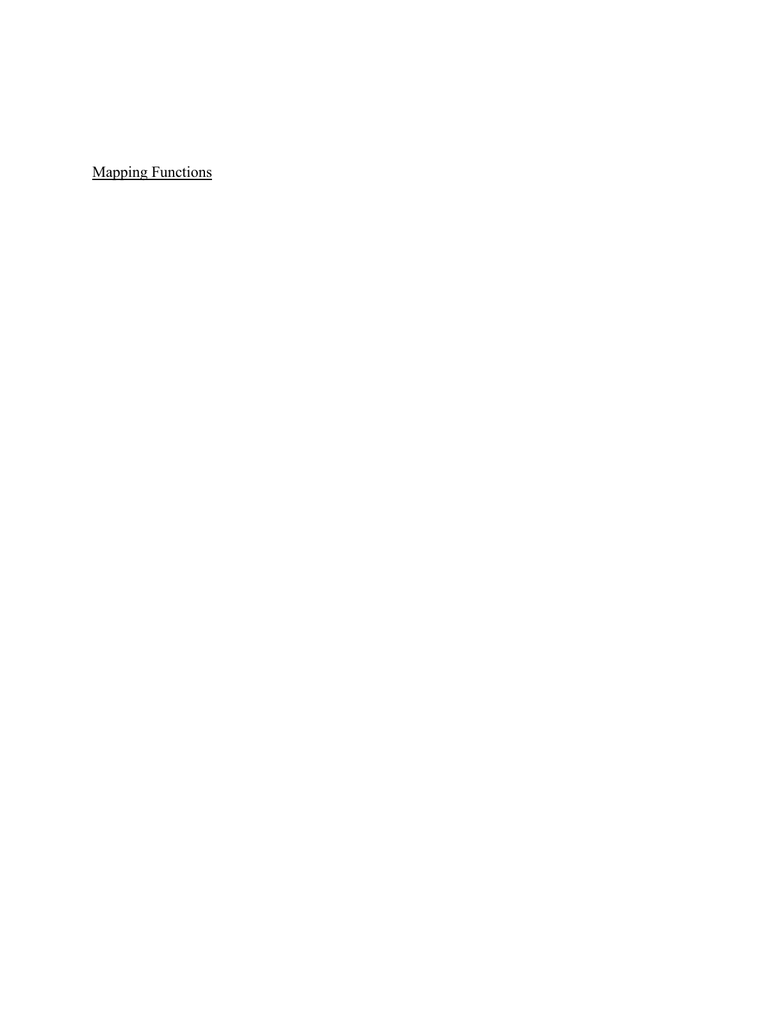Textbook Notes (280,000)
US (110,000)
UD (1,000)
CGSC (70)
CGSC170 (20)
Chapter 8

# CGSC170 Chapter Notes - Chapter 8: Boolean Function, George Boole, Sentence Clause Structure

Department
Cognitive Science
Course Code
CGSC170
Professor
Kaja Jasinka
Chapter
8

This preview shows half of the first page. to view the full 3 pages of the document.Single Layer Networks and Boolean functions
Mapping Functions
Functions are understood strictly mathematically in this sense
Ex-1-
Two numbers as inputs yields a third number as the output
Output is the sum of two inputs
Ex-2
a set of numbers
Domain
Another set of numbers
Range
A mapping function maps each item from the domain onto exactly one
item from the range
1:1 ratio
No item from the domain gets mapped more than once
No item from the domain gets mapped to more than one item in the
range
Functions are single valued
Ex3- square roots of numbers are not found through functions because for every
positive number there is 2 square roots
Two number in the domain may b mapped to a single item in a range